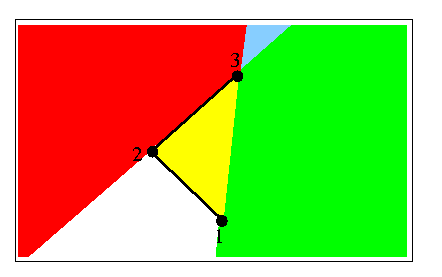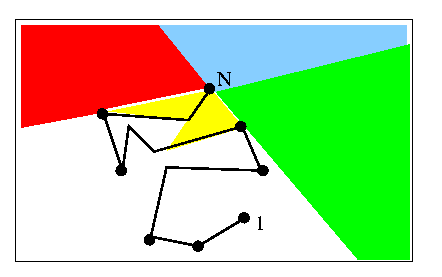Melkman 1987Best algorithm

Melkman's algorithm  is considered to be the best convex hull algorithm for simple polygons. What distinguishes it from all the rest is that it is actually an on-line algorithm. It computes the hull as points are being entered (notice that all other algorithms require finding an extreme point first). This means that the ordering of vertices (CW or CCW) does not need to be known either. The algorithm is only a few lines long, and his entire article is printed on two sides of a single page. Melkman uses the same logic as Lee, Graham and Yao (and others), i.e. he partitions the plane into regions that the next vertex could be. The difference is that he allows vertices to be removed on both sides of the forming chain. Melkman acknowledges that a similar approach to his was taken independently by Tor and Middleditch , who were working on the convex decomposition of a simple polygon.

Very informally, here's what the algorithm does:

It takes the first three vertices (starting anywhere) and sets up the current convex hull, i.e. the triangle formed. In the picture below, they happen to form a right turn (clockwise). Vertices are stored in a double ended queue (deque) : (bottom) 3-1-2-3 (top). Notice that if we take the last three vertices of the bottom in the order 2,1,3 we get a left turn. If we take the last three vertices from the top, in the order 1,2,3 we get a right turn. This is a property that we wish to maintain: in general as we read the deque from bottom to top, we get the hull in clockwise order, and as we read from top to bottom we get a counterclockwise order.

Now the next vertex, V, could be in the red/green/blue/yellow regions.If V is in the yellow region, ignore it and all following vertices until one emerges into the other regions. Call the emerging vertex V. If V is not yellow, we must add it to the deque on both sides, because it will be on the current hull. However we must ensure that we preserve our clockwise/counterclockwise property if this is to be done:

If V is in the red region, then 2,3,V form a left turn. Backtrack/delete vertices from the top of the deque (i.e. 3, then maybe 2, etc), until a right turn is formed by the last 3 vertices.

If V is in the green region, then 1,3,V form a right turn. Backtrack/delete vertices from the bottom of the deque (i.e. 3, then maybe 1, etc), until a left turn is formed by the last 3 vertices.
Notice that this case is symmetric to the red region.

If blue, follow the instructions for both red and green.

Now the deque structure is correct and we can process the next point.

The figure below shows these regions in a more general case. Vertices on the hull have circles on them. (N) is the last vertex added. The next vertex could be anywhere in the colored regions. Note that you can find these regions by looking at N, and its neighbors on the hull, which are conveniently represented at the two ends of the deque.Check out Pierre Lang's project (including an applet) on Melkman's algorithm. To enter a simple polygon in his applet, make sure that the line between the yellow dot and the mouse tip does not intersect any of the lines already drawn, except the line segment between the yellow and orange dots. In other words, imagine that this segment doesn't exist.

previous : Werner 1986.          next : Chen 1989.